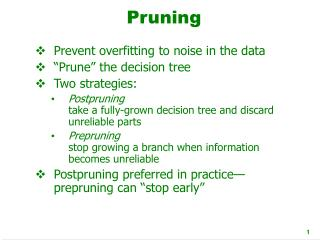DownloadDownload PresentationPruning

# Pruning

Télécharger la présentation## Pruning

- - - - - - - - - - - - - - - - - - - - - - - - - - - E N D - - - - - - - - - - - - - - - - - - - - - - - - - - -
##### Presentation Transcript

1. Pruning • Prevent overfitting to noise in the data • “Prune” the decision tree • Two strategies: • Postpruningtake a fully-grown decision tree and discard unreliable parts • Prepruningstop growing a branch when information becomes unreliable • Postpruning preferred in practice—prepruning can “stop early”

2. Prepruning • Based on statistical significance test • Stop growing the tree when there is no statistically significant association between any attribute and the class at a particular node • Most popular test: chi-squared test • ID3 used chi-squared test in addition to information gain • Only statistically significant attributes were allowed to be selected by information gain procedure

3. Early stopping • Pre-pruning may stop the growth process prematurely: early stopping • Classic example: XOR/Parity-problem • No individual attribute exhibits any significant association to the class • Structure is only visible in fully expanded tree • Prepruning won’t expand the root node • But: XOR-type problems rare in practice • And: prepruning faster than postpruning

4. Postpruning • First, build full tree • Then, prune it • Fully-grown tree shows all attribute interactions • Problem: some subtrees might be due to chance effects • Two pruning operations: • Subtree replacement • Subtree raising • Possible strategies: • error estimation • significance testing • MDL principle

5. Subtreereplacement • Bottom-up • Consider replacing a tree only after considering all its subtrees

6. Subtree raising • Delete node • Redistribute instances • Slower than subtree replacement (Worthwhile?)

7. Estimating error rates • Prune only if it reduces the estimated error • Error on the training data is NOT a useful estimator(would result in almost no pruning) • Use hold-out set for pruning(“reduced-error pruning”) • C4.5’s method • Derive confidence interval from training data • Use a heuristic limit, derived from this, for pruning • Standard Bernoulli-process-based method • Shaky statistical assumptions (based on training data)

8. C4.5’s method • Error estimate for subtree is weighted sum of error estimates for all its leaves • Error estimate for a node: • If c = 25% then z = 0.69 (from normal distribution) • f is the error on the training data • N is the number of instances covered by the leaf

9. Example Example f = 5/14 e = 0.46e < 0.51so prune! f=0.33 e=0.47 f=0.5 e=0.72 f=0.33 e=0.47 (0.47*6 + 0.72 * 2 + 0.47 * 6)/14=0.51 Combined using ratios 6:2:6 gives 0.51